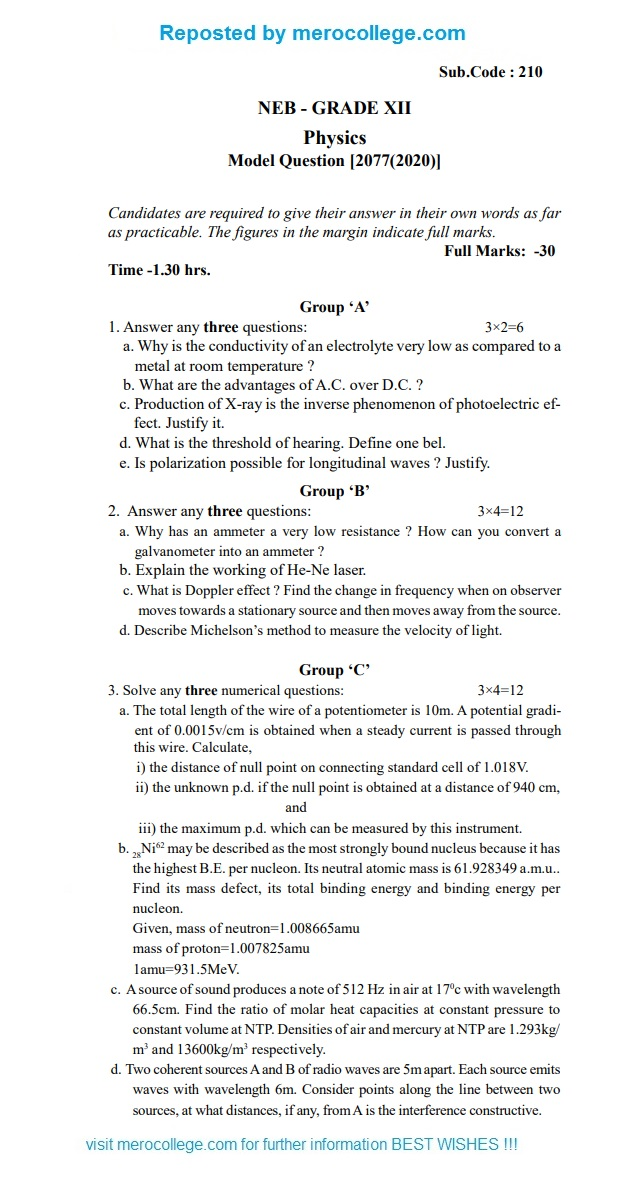# NEB 12 Model Questions 2020 Physics# NEB XII Model Questions 2020 Physics

NEB 12 Model Questions 2020 for Physics has been provided by National Examination Board, Nepal (NEB) for it’s upcoming Class XII Examinations.Sub.Code : 210

Physics

Model Question [2077(2020)]

Candidates are required to give their answer in their own words as far as practicable. The figures in the margin indicate full marks.

Full Marks: -30

Time -1.30 hrs.

Group ‘A’

1. Answer any three questions: 3×2=6

a. Why is the conductivity of an electrolyte very low as compared to a metal at room temperature ?

1. What are the advantages of A.C. over D.C.?
2. Productionof X-ray is the inverse phenomenon of photoelectric ef- fect. Justify
3. What is the threshold of hearing. Define one
4. Is polarization possible for longitudinal waves ?

Group ‘B’

1. Why has an ammeter a very low resistance ? How can you convert a galvanometer into an ammeter?

b. Explain the working of He-Ne laser.

1. Whatis Doppler effect ? Find the change in frequency when on observer moves towards a stationary source and then moves away from the
2. Describe Michelson’s method to measure the velocity of

Group ‘C’

1. Solve any threenumerical questions: 3×4=12
1. The total length of the wire of a potentiometer is 10m. A potential gradi- ent of 0.0015v/cm is obtained when a steady current is passedthrough

this wire. Calculate,

1. the distance of null point on connecting standard cell of018V.
2. the unknown p.d. if the null point is obtained at a distance of 940cm,

and

• the maximum p.d. which can be measured by this
1. Ni62may be described as the most strongly bound nucleus because it has the highest E. per nucleon. Its neutral atomic mass is 61.928349 a.m.u.. Find its mass defect, its total binding energy and binding energy per nucleon.

Given, mass of neutron=1.008665amu mass of proton=1.007825amu 1amu=931.5MeV.

1. Asource of sound produces a note of 512 Hz in air at 170c with wavelength 66.5cm. Find the ratio of molar heat capacities at constant pressure to constant volume at  Densities of air and mercury at NTP are 1.293kg/ m3 and 13600kg/m3 respectively.
2. Twocoherent sources A and B of radio waves are 5m  Each source emits waves with wavelength 6m. Consider points along the line between two sources, at what distances, if any, from A is the interference constructive.

Please visit for more detail :